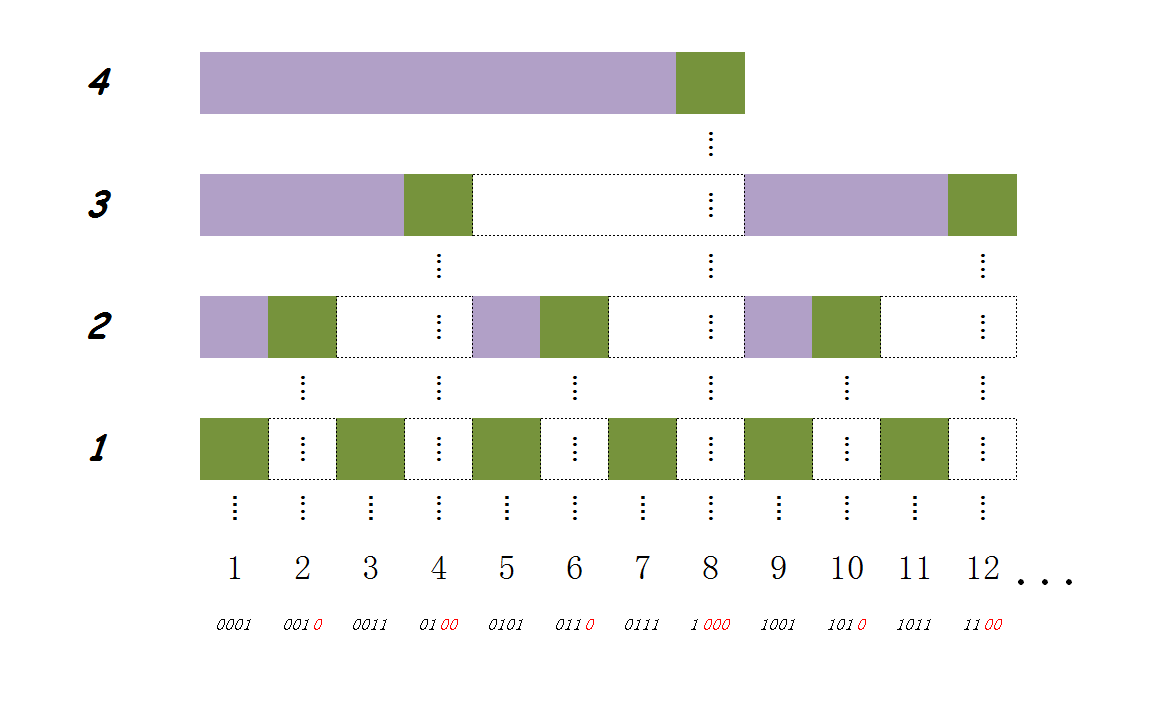# lowbit函数

• 还记得 剑指Offer66题之每日6题 - 第二天中的第五题中讲过的如何消掉最后一位1吗？我们就是先消掉最后一位1，然后再用原数减去消掉最后一位1后的数，答案就是lowbit(x)的结果；

• 第二种方法就是计算机组成原理课上老师教过我们求负数的补码的简便方法：把这个数的二进制写出来，然后从右向左找到第一个1(这个1就是我们要求的结果，但是现在表示不出来，后来的操作就是让这个1能表示出来)，这个1不要动和这个1右边的二进制不变，左边的二进制依次取反，这样就求出的一个数的补码，说这个方法主要是让我们理解一个负数的补码在二进制上的特征，然后我们把这个负数对应的正数与该负数与运算一下，由于这个1的左边的二进制与正数的原码对应的部分是相反的，所以相与一定都为0,；由于这个1和这个1右边的二进制都是不变的，因此，相与后还是原来的样子，故，这样搞出来的结果就是lowbit(x)的结果。

int lowbit(x)
{
return x - (x & (x - 1));
}
int lowbit(x)
{
return x & -x;
}

# 树状数组的思想

$\begin{array}{}\text{(2)}& \sum _{i=1}^{6}=\left(ar{r}_{1}+ar{r}_{2}+ar{r}_{3}+ar{r}_{4}\right)+\left(ar{r}_{5}+ar{r}_{6}\right)\end{array}$

# 树状数组的实现

## 修改• 后缀和的长度是2的幂；
• 上一层后缀和的长度是下一层后缀和长度的两倍；
• 下一层后缀和只要补上自己后缀和的长度就可以得到上面层的后缀和(图中的虚框框)，注意，是上面的后缀和，而不是上一层的后缀和，这个性质就是更新操作的依据；
• 最后一位1右边有多少个0(可以用$lo{g}_{2}\left(lowbit\left(x\right)\right)$$log_2(lowbit(x))$表示)就表示这一层有多少个直系子层(子层的意思就是这一层的和包含下面某一层的和)。

# 树状数组的代码

## 查询前缀和

int sum(int x, ArrayInt c, int n)
{
int ret = 0;
for ( ; x > 0; ret += c[x], x -= lowbit(x));
return ret;
}

## 更新后缀和

void update(int x, int val, ArrayInt c, int n)
{
for ( ; x <= n; c[x] += val, x += lowbit(x));
}

## 完整代码

### Code:

#include <stdio.h>
#include <stdlib.h>
#include <string.h>

#define lowbit(x) ((x) & -(x))

typedef int *ArrayInt;

int sum(int x, ArrayInt c, int n)
{
int ret = 0;
for ( ; x > 0; ret += c[x], x -= lowbit(x));
return ret;
}

void update(int x, int val, ArrayInt c, int n)
{
for ( ; x <= n; c[x] += val, x += lowbit(x));
}

int main()
{
int n, i, m;
ArrayInt arr = NULL, c = NULL;
char ope;

scanf("%d", &n);
arr = (ArrayInt)malloc((n + 1) * sizeof(int));
c = (ArrayInt)malloc((n + 1) * sizeof(int));
memset(c, 0, (n + 1) * sizeof(int));
for (i = 1; i <= n; scanf("%d", arr + i), update(i, arr[i], c, n), ++i);

for (scanf("%d", &m) ; m--; ) {
int L, R;
scanf("%s%d%d", ope, &L, &R);

switch (ope) {
case 'F' :
printf("%d\n", sum(R, c, n) - sum(L - 1, c, n));
break;
default :
update(L, R - arr[L], c, n);
arr[L] = R;
break;
}
}
return 0;
}

# 总结